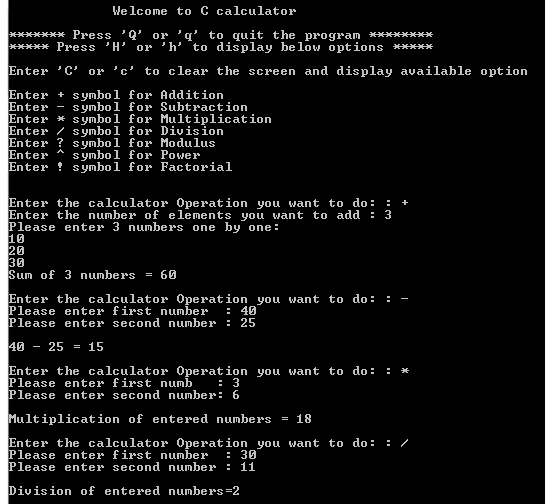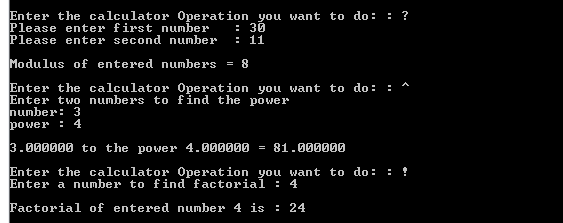# C – Code for Calculator Application

A sample C programming code for a real time calculator application program is given below. This program will perform the below calculator operations.

2. Subtraction
3. Multiplication
4. Division
5. Modulus
6. Power
7. Factorial

In below c programming code example, the user is prompted to choose the operations(i.e. addition, subtraction etc)  to be performed and then prompted to key in the values which are used to perform the operations. Then, the result will be shown as output to the user.

#### Sample calculator Output using C programming code:Continue on “C code for bank application”….

Continue on “C interview questions and answers”….